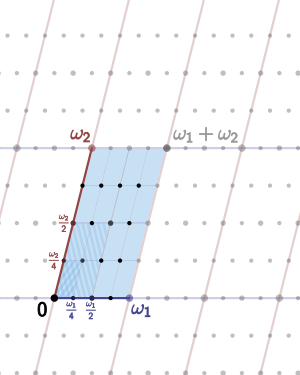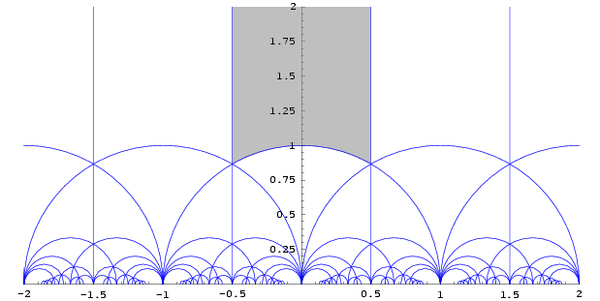# Fundamental domain

Given a topological space and a group acting on it, the images of a single point under the group action form an orbit of the action. A fundamental domain is a subset of the space which contains exactly one point from each of these orbits. It serves as a geometric realization for the abstract set of representatives of the orbits.

There are many ways to choose a fundamental domain. Typically, a fundamental domain is required to be a connected subset with some restrictions on its boundary, for example, smooth or polyhedral. The images of a chosen fundamental domain under the group action then tile the space. One general construction of fundamental domains uses Voronoi cells.

## Hints at general definitionA lattice in the complex plane and its fundamental domain, with quotient a torus.

Given an action of a group G on a topological space X by homeomorphisms, a fundamental domain (also called fundamental region) for this action is a set D of representatives for the orbits. It is usually required to be a reasonably nice set topologically, in one of several precisely defined ways. One typical condition is that D is almost an open set, in the sense that D is the symmetric difference of an open set in G with a set of measure zero, for a certain (quasi)invariant measure on X. A fundamental domain always contains a free regular set U, an open set moved around by G into disjoint copies, and nearly as good as D in representing the orbits. Frequently D is required to be a complete set of coset representatives with some repetitions, but the repeated part has measure zero. This is a typical situation in ergodic theory. If a fundamental domain is used to calculate an integral on X/G, sets of measure zero do not matter.

For example, when X is Euclidean space Rn of dimension n, and G is the lattice Zn acting on it by translations, the quotient X/G is the n-dimensional torus. A fundamental domain D here can be taken to be [0,1)n, which differs from the open set (0,1)n by a set of measure zero, or the closed unit cube [0,1]n, whose boundary consists of the points whose orbit has more than one representative in D.

## Examples

Examples in the three-dimensional Euclidean space R3.

• for n-fold rotation: an orbit is either a set of n points around the axis, or a single point on the axis; the fundamental domain is a sector
• for reflection in a plane: an orbit is either a set of 2 points, one on each side of the plane, or a single point in the plane; the fundamental domain is a half-space bounded by that plane
• for inversion in a point: an orbit is a set of 2 points, one on each side of the center, except for one orbit, consisting of the center only; the fundamental domain is a half-space bounded by any plane through the center
• for 180° rotation about a line: an orbit is either a set of 2 points opposite to each other with respect to the axis, or a single point on the axis; the fundamental domain is a half-space bounded by any plane through the line
• for discrete translational symmetry in one direction: the orbits are translates of a 1D lattice in the direction of the translation vector; the fundamental domain is an infinite slab
• for discrete translational symmetry in two directions: the orbits are translates of a 2D lattice in the plane through the translation vectors; the fundamental domain is an infinite bar with parallelogrammatic cross section
• for discrete translational symmetry in three directions: the orbits are translates of the lattice; the fundamental domain is a primitive cell which is e.g. a parallelepiped, or a Wigner-Seitz cell, also called Voronoi cell/diagram.

In the case of translational symmetry combined with other symmetries, the fundamental domain is part of the primitive cell. For example, for wallpaper groups the fundamental domain is a factor 1, 2, 3, 4, 6, 8, or 12 smaller than the primitive cell.

## Fundamental domain for the modular groupEach triangular region is a free regular set of H/Γ; the grey one (with the third point of the triangle at infinity) is the canonical fundamental domain.

The diagram to the right shows part of the construction of the fundamental domain for the action of the modular group Γ on the upper half-plane H.

This famous diagram appears in all classical books on modular functions. (It was probably well known to C. F. Gauss, who dealt with fundamental domains in the guise of the reduction theory of quadratic forms.) Here, each triangular region (bounded by the blue lines) is a free regular set of the action of Γ on H. The boundaries (the blue lines) are not a part of the free regular sets. To construct a fundamental domain of H/Γ, one must also consider how to assign points on the boundary, being careful not to double-count such points. Thus, the free regular set in this example isThe fundamental domain is built by adding the boundary on the left plus half the arc on the bottom including the point in the middle:The choice of which points of the boundary to include as a part of the fundamental domain is arbitrary, and varies from author to author.

The core difficulty of defining the fundamental domain lies not so much with the definition of the set per se, but rather with how to treat integrals over the fundamental domain, when integrating functions with poles and zeros on the boundary of the domain.